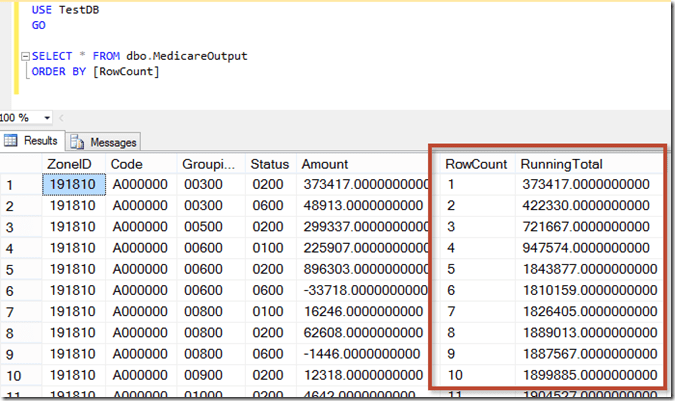# Row Numbers and Running Totals in SSISDuring data load or transformation processes, capturing a distinct row number for incoming data can be beneficial for the ETL process itself, as well as for use in the destination database. Having an arbitrary, incrementing row number assigned to each row can help to determine the order in which the rows of data were processed, and can provide a unique value (for the in-process data set, at least) that can be used for any updates downstream. Similarly, calculating a running total on a numerical column is often useful for in-process sets of data. Think about things like bank balances, where you might derive the account status (Good or Overdrawn) based on the account balance at that exact point in time. Running totals can sometimes be used to check for data quality issues prior to loading, such as flagging duplicate IDs or looking for business rule violations.

In this blog post, I’ll demonstrate how you can easily add row number and running total functionality to a SQL Server Integration Services data flow. By using just a few lines of code in the script component, you can add in a row number and a running total column that can be loaded to your destination.

One word of caution on this approach: If you don’t have to use it, don’t use it. It’s not that this is a bad approach, but, as I keep saying, there is a tool for every job. If you need to capture row numbers or running totals while running an ad-hoc query against a relational database, check first to see if you can use windowing functions in the source query. Most of the major relational database engines have some form of windowing functions. If you can easily get that information in the source query, do so – you’ll end up with a simpler solution. However, if you are dealing with nonrelational data (plain text, XML, Excel, etc.) and you require this type of functionality during ETL, this is a very simple and effect method for getting that information.

### Creating Row Numbers and Running Totals in SSIS

For this example, I’m going to use a large flat file as a source, writing that data out to a SQL Server destination table. I’ll modify the package shown below to capture the row number and calculate the running total of the transaction amount.Adding a row number and a running total column to an Integration Services data flow is actually a fairly simple operation, though it does require some coding. The easiest method to accomplish this directly in the data flow is to use a script component as a transformation, adding in new columns on the script component output to store the row number and running total values. For this example,  I added a script component transformation and connected it to the flat file source (but not yet connected the output – that’ll come next). Shown below is the output details of the script component, where I have added the two additional columns to capture row number and the running total.It is important to note that this script transformation is working synchronously, which means that for each input row, there will be exactly one output row with the same metadata. In a synchronous script component, I can add new columns to the output but cannot delete or modify any existing columns. Show above, I’ve simply added two new columns to the output, which will be used to store the row number and running total values. The script component transformation will run synchronously by default.

In the code of the script transformation, two new variables (native C# variables, not SSIS variables) should be created to store the in-process row number and running total values. These variables should be declared within the class declaration but outside of any function. As shown below, I have created those two variables and instantiated them (set to zero) in the PreExecute() function.

```public class ScriptMain : UserComponent
{

// Create the variables that will track the running values
int rowCount;
decimal runningTotal;

public override void PreExecute()
{
base.PreExecute();

// Initialize the running values
rowCount = 0;
runningTotal = 0;
}

...
}
```

Next up, I’ll add the code to update those variables for each row processed. To do so, I’ll be modifying the Input0_ProcessInputRow() function (where Input0 is the name of the input). As shown below, I’m arbitrarily incrementing the row number variable, and adding the transaction amount value for the current row to the running total variable.

```public override void Input0_ProcessInputRow(Input0Buffer Row)
{
// Increment the running values
rowCount++;
runningTotal += Row.Amount;
}```

Finally, I will set the value of those new columns I added (see the above screen shot) using the current value of the C# variables for row number and running total.

```public override void Input0_ProcessInputRow(Input0Buffer Row)
{
// Increment the running values
rowCount++;
runningTotal += Row.Amount;

// Set the running values in the current row
Row.RowCount = rowCount;
Row.RunningTotal = runningTotal;
}```

The only thing left to do is to connect the output of the script component to the input of the database destination, making sure to connect the two new calculated columns to the appropriate columns in the destination.As shown below, the two new columns will now appear in my output, showing both the row number and the running transaction amount total.### Conclusion

Using the design pattern above, I have added both row numbers and running totals to the SSIS data flow, using only a few lines of relatively simple code. As I mentioned, this is a good option if you need this functionality for a data source that does not provide these types of windowing functions, such as text, XML, or similar data sources. Using row numbers and running totals in SSIS data flows